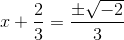# High School Math : Completing the Square

## Example Questions

### Example Question #31 : Solving Quadratic Equations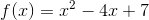Find the vertex of the parabola by completing the square.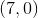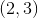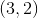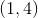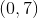Explanation:

To find the vertex of a parabola, we must put the equation into the vertex form: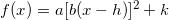The vertex can then be found with the coordinates (h, k).

To put the parabola's equation into vertex form, you have to complete the square. Completing the square just means adding the same number to both sides of the equation -- which, remember, doesn't change the value of the equation -- in order to create a perfect square.Put all of theterms on one side: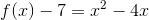Now we know that we have to add something to both sides in order to create a perfect square: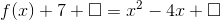In this case, we need to add 4 on both sides so that the right-hand side of the equation factors neatly.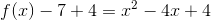Now we factor:Once we isolate, we have the equation in vertex form: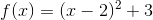Thus, the parabola's vertex can be found at.

### Example Question #32 : Solving Quadratic Equations

Complete the square: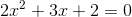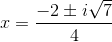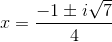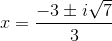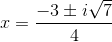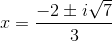Explanation:

Begin by dividing the equation byand subtractingfrom each side: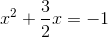Square the value in front of theand add to each side: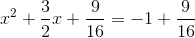Factor the left side of the equation: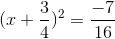Take the square root of both sides and simplify: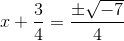### Example Question #33 : Solving Quadratic Equations

Use factoring to solve the quadratic equation: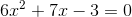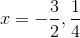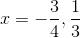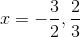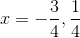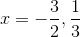Explanation:

Factor and solve: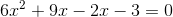Factor like terms: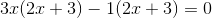Combine like terms: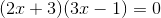### Example Question #34 : Solving Quadratic Equations

Complete the square: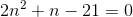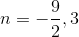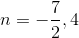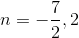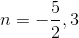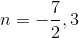Explanation:

Begin by dividing the equation byand adding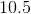to each side: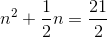Square the value in front of theand add to each side: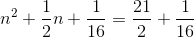Factor the left side of the equation: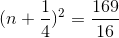Take the square root of both sides and simplify: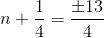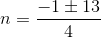### Example Question #35 : Solving Quadratic Equations

Complete the square: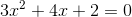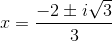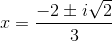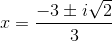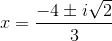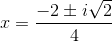Explanation:

Begin by dividing the equation byand subtractingfrom each side: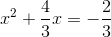Square the value in front of theand add to each side: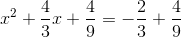Factor the left side of the equation: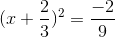Take the square root of both sides and simplify: